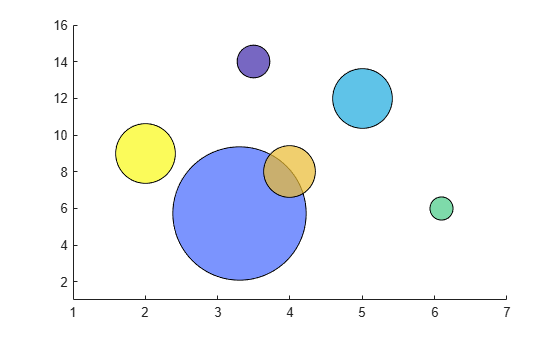# lines

Lines colormap array

•## Syntax

``c = lines``
``c = lines(m)``

## Description

````c = lines` returns the lines colormap as a three-column array with the same number of rows as the colormap for the current figure. If no figure exists, then the array contains 256 rows. Each row in the array contains the red, green, and blue intensities for a specific color. The intensities are in the range [0,1], and the color scheme matches the default `ColorOrder` property of the `Axes`. Thus, you can use this colormap to make your chart colors match the output of the `plot` function.```

example

````c = lines(m)` returns the colormap with `m` colors.```

## Examples

collapse all

Create a scatter plot using the default colors.

```x = [3.5 3.3 5 6.1 4 2]; y = [14 5.7 12 6 8 9]; sz = 100*[6 100 20 3 15 20]; c = [1 2 3 4 5 6]; scatter(x,y,sz,c,'filled','MarkerEdgeColor','k','MarkerFaceAlpha',.7); xlim([1 7]); ylim([1 16]);```Get the lines colormap array with six entries. Then replace the colormap in the scatter plot.

```c = lines(6); colormap(c);```## Input Arguments

collapse all

Number of colors, specified as a positive number. The default value of `m` is the number of colors in the current figure's colormap. If no figure exists, the default value is 256.

Data Types: `single` | `double`

## Version History

Introduced before R2006a

expand all